Home  |  Contact
 DESENMASCARANDO LAS FALSAS DOCTRINAS

 What’s NewMessage Board
Image Gallery
Files and Documents
Polls and Test
Member List
YHWH (DIOS PADRE) EL UNICO DIOS
JESUCRISTO NUESTRO MESIAS JUDIO
LOS DIEZ MANDAMIENTOS DE LA BIBLIA
MEJORE SU CARACTER Y SU VIDA
BIBLIAS/CONCORDANCIA/LIBROS
MAYOR ENEMIGO DEL HOMBRE ES UNO MISMO
¿LA TORA ES MACHISTA? -MENSAJE ESOTERICO Y EXOTERICO
¿ES INMORTAL EL ALMA?- FALACIA DE LA ENCARNACION Y REENCARNACION
EL ISLAM TIENE ORIGEN UNITARIO ADOPCIONISTA
ANTIGUO TESTAMENTO-ESTUDIO POR VERSICULOS
NUEVO TESTAMENTO-ESTUDIOS POR VERSICULOS
NUEVO TESTAMENTO II-ESTUDIOS POR VERSICULOS
NUEVO TESTAMENTO III-ESTUDIOS POR VERSICULOS
CRISTO NO TUVO PREEXISTENCIA
¿QUE ES EL ESPIRITU SANTO?

Tools

MATEMATICAS: EL RELIGIOSO "PROGRE" SE OLVIDO QUE YHWH ES SINONIMO DE MATEMATICAS
Choose another message boardPrevious subject  Next subjectReply Message 1 of 90 on the subject
 From: BARILOCHENSE6999  (Original message) Sent: 22/12/2020 20:09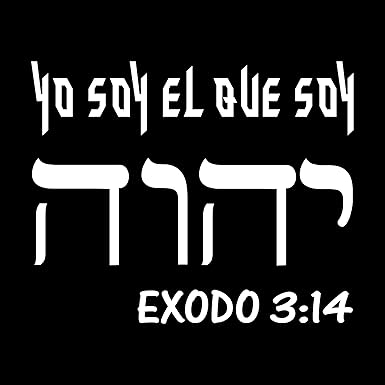FirstPrevious  61 to 75 of 90  NextLastReply Message 61 of 90 on the subject
 From: BARILOCHENSE6999 Sent: 22/07/2021 23:48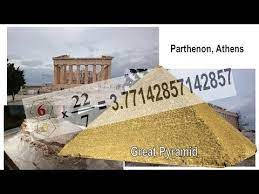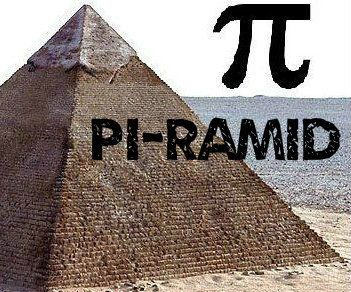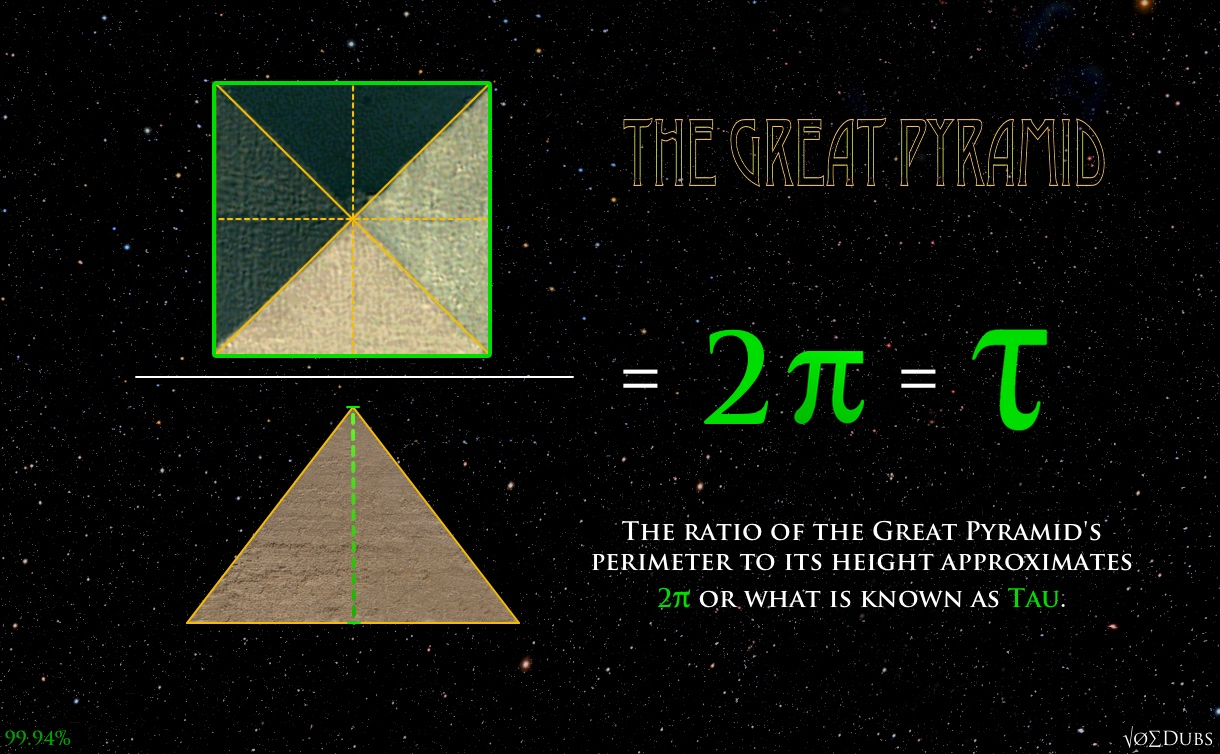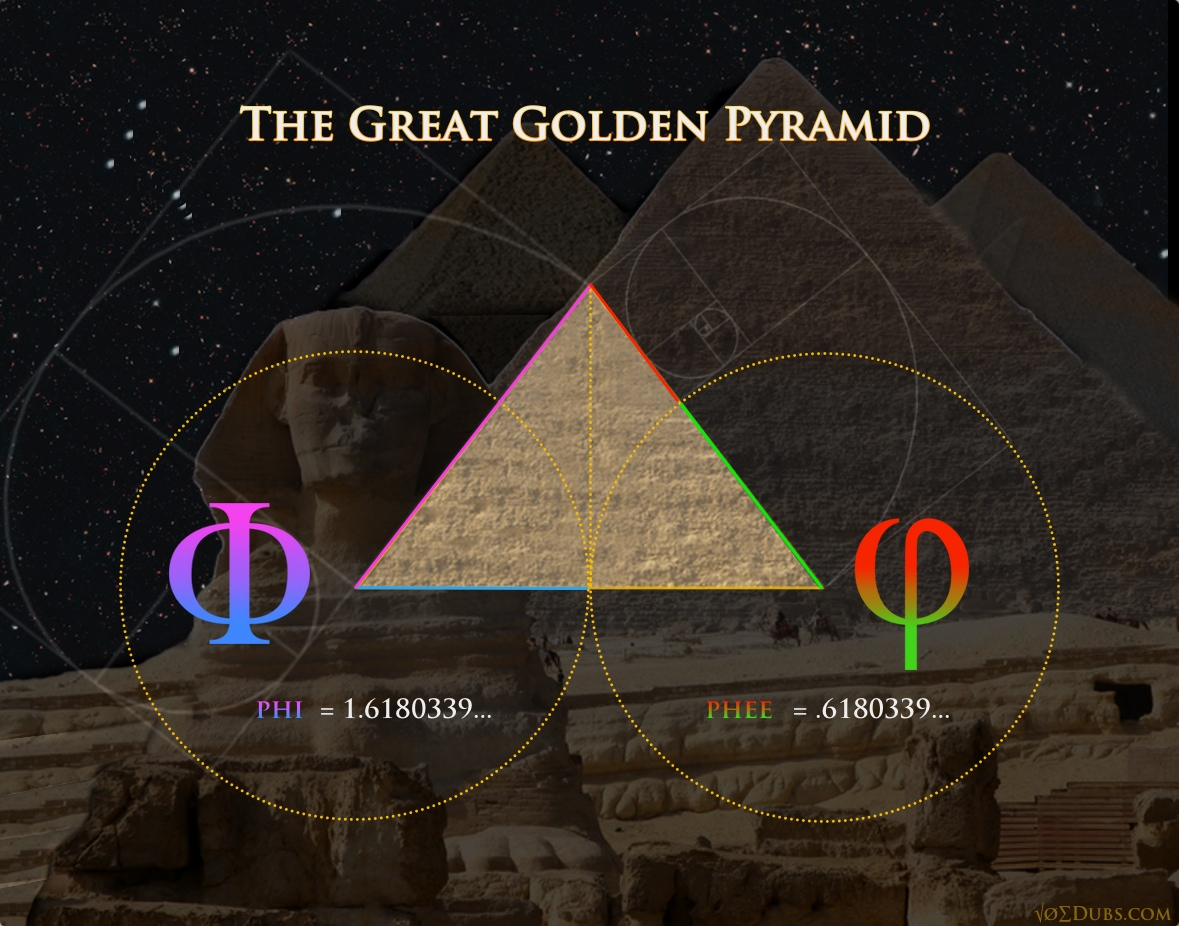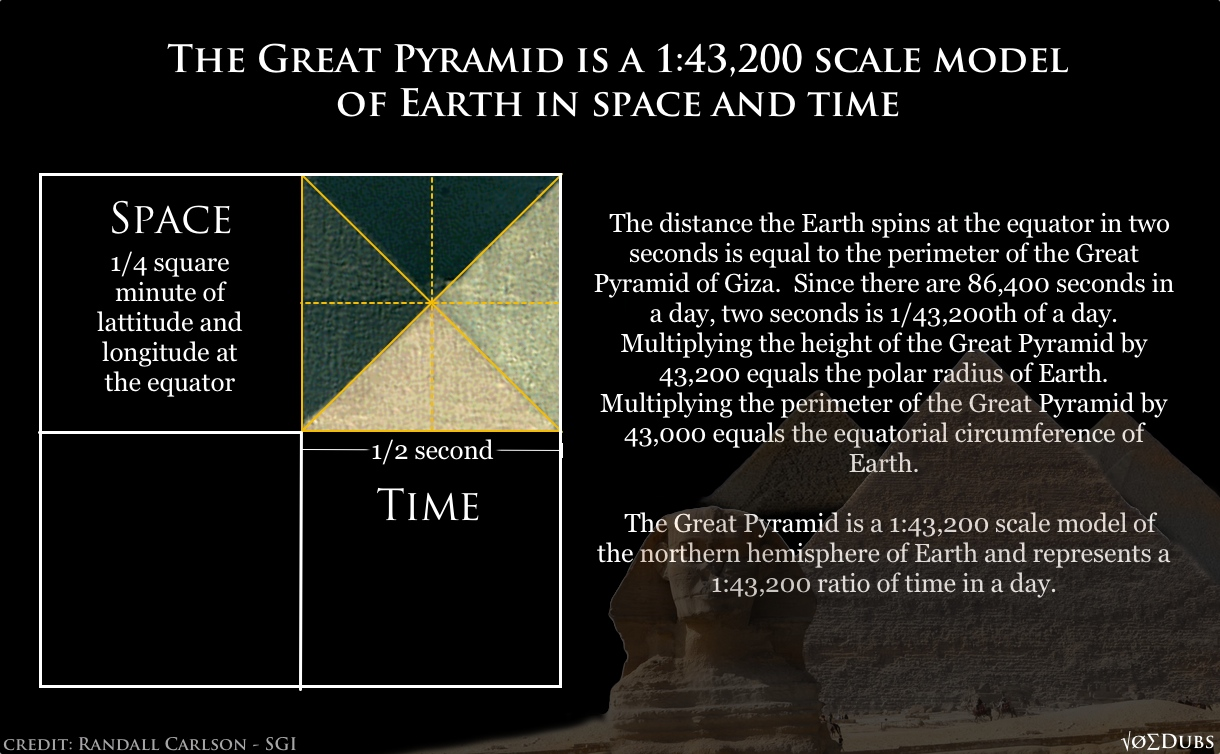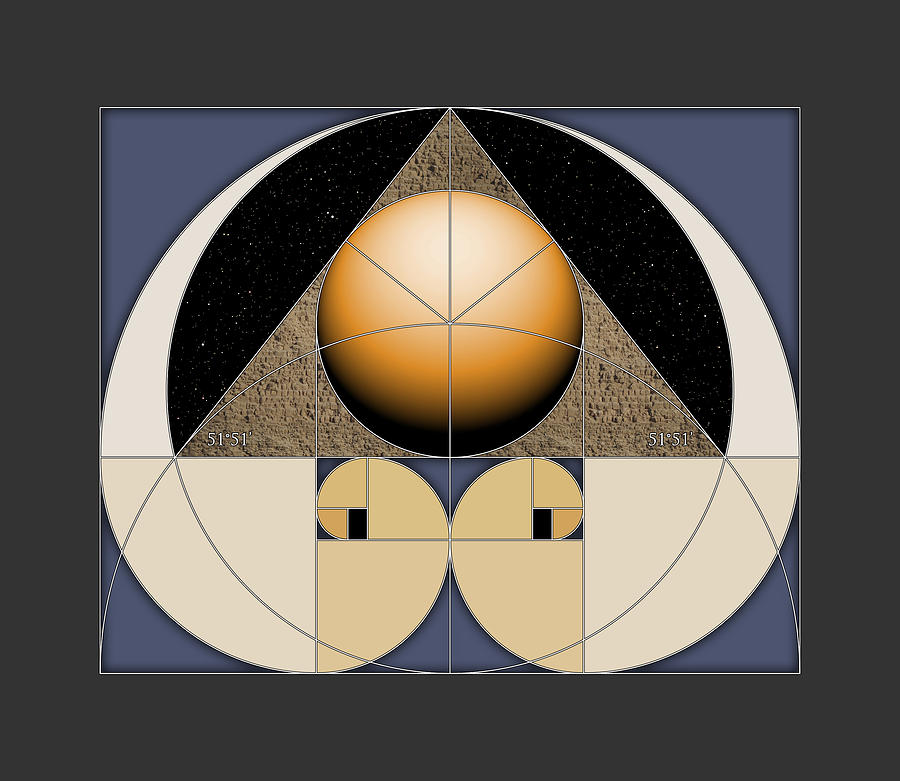Reply Message 62 of 90 on the subject
 From: BARILOCHENSE6999 Sent: 25/07/2021 19:37

 Reply Message 63 of 90 on the subject
 From: BARILOCHENSE6999 Sent: 25/07/2021 23:02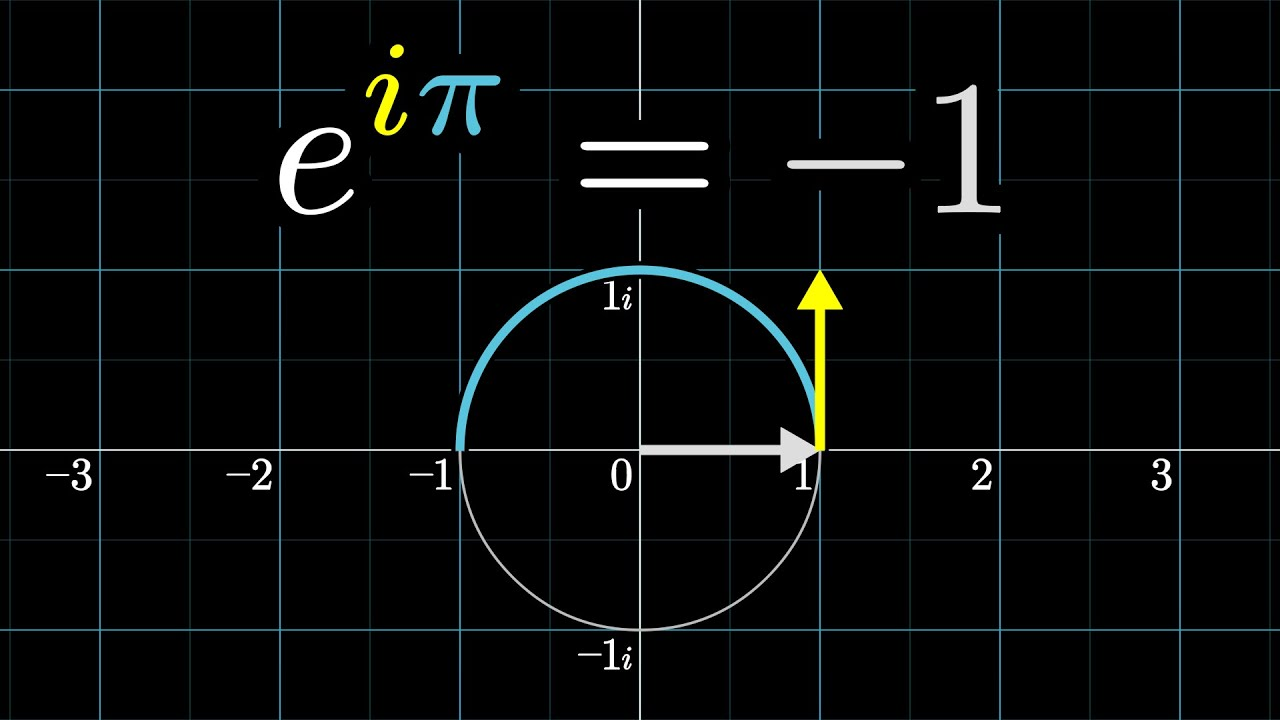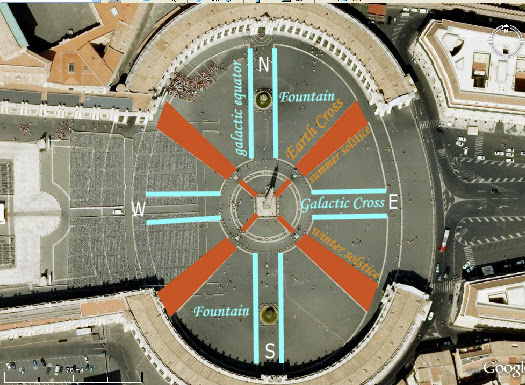Reply Message 64 of 90 on the subject
 From: BARILOCHENSE6999 Sent: 27/07/2021 12:35

 Reply Message 65 of 90 on the subject
 From: BARILOCHENSE6999 Sent: 03/08/2021 23:05Respuesta Ocultar Mensaje Eliminar Mensaje Mensaje 287 de 287 en el tema"Harmonic 360 of The Circle"The ancients selected the division of 360 degrees for a specific reason.(Observe the outer ring of this complex Ying-Yang Chart having specifically 360 divisions).The factors of the number 36 are: 1, 2, 3, 4, 6, 9, 12, 18, 36indicating that it has an unusually large range of divisors and therefore more friends with other numbers.…So irrational! Euler's identity = e ^ (ix) = cos x + i sin xAs cos (pi) = -1 and sin (pi) = 0,e^(i.pi) = -1 + i.0 = -1Hence, e^(i.pi) + 1 = 0Unfortunately, it had to be said, this is very incorrect.With Circle's diameter, also equal to (A * G) = Real_Pi = 3.144605511...A = Base Cathetus = Diameter / G = 1.94347308702659Nowhere close to Phi....And B = Opposite Cathetus = A * SQRT (G) = 2.47213595499959Reply Message 66 of 90 on the subject
 From: BARILOCHENSE6999 Sent: 03/08/2021 23:21Respuesta Ocultar Mensaje Eliminar Mensaje Mensaje 23 de 23 en el tema
 In celestial mechanics Mercury completes one cycle every 116 days. This means that in one solar year, Mercury will complete 365÷116 or 3.14 cycles. Because of this decimal interval the ancients observed Mercury in 7-year periods. Every 7 years, Mercury completes 22 conjunction cycles (because pi is approximately equal to 22÷7, this ratio is extremely precise with only an hour difference!).Jain 108The BOOK Of PHI, vol 8, True Value Of Pi = JainPi = 3.144...http://www.jainmathemagics.com/product/134/default.asp?

 Reply Message 67 of 90 on the subject
 From: BARILOCHENSE6999 Sent: 08/08/2021 13:13

 Reply Message 68 of 90 on the subject
 From: BARILOCHENSE6999 Sent: 14/08/2021 01:57Reply Message 69 of 90 on the subject
 From: BARILOCHENSE6999 Sent: 14/08/2021 11:25

 Reply Message 70 of 90 on the subject
 From: BARILOCHENSE6999 Sent: 16/08/2021 10:37

 Reply Message 71 of 90 on the subject
 From: BARILOCHENSE6999 Sent: 07/09/2021 00:47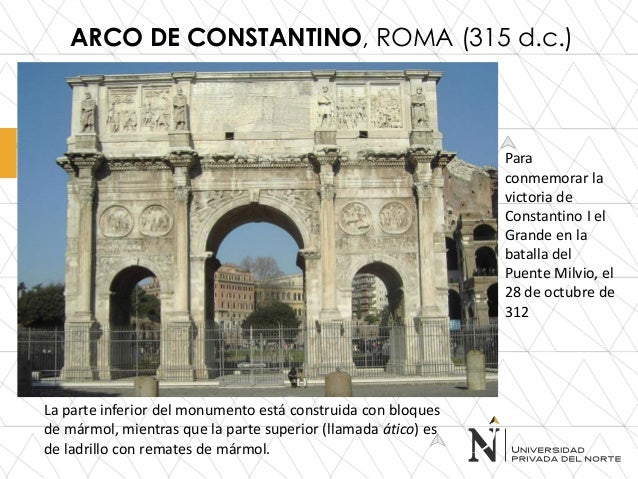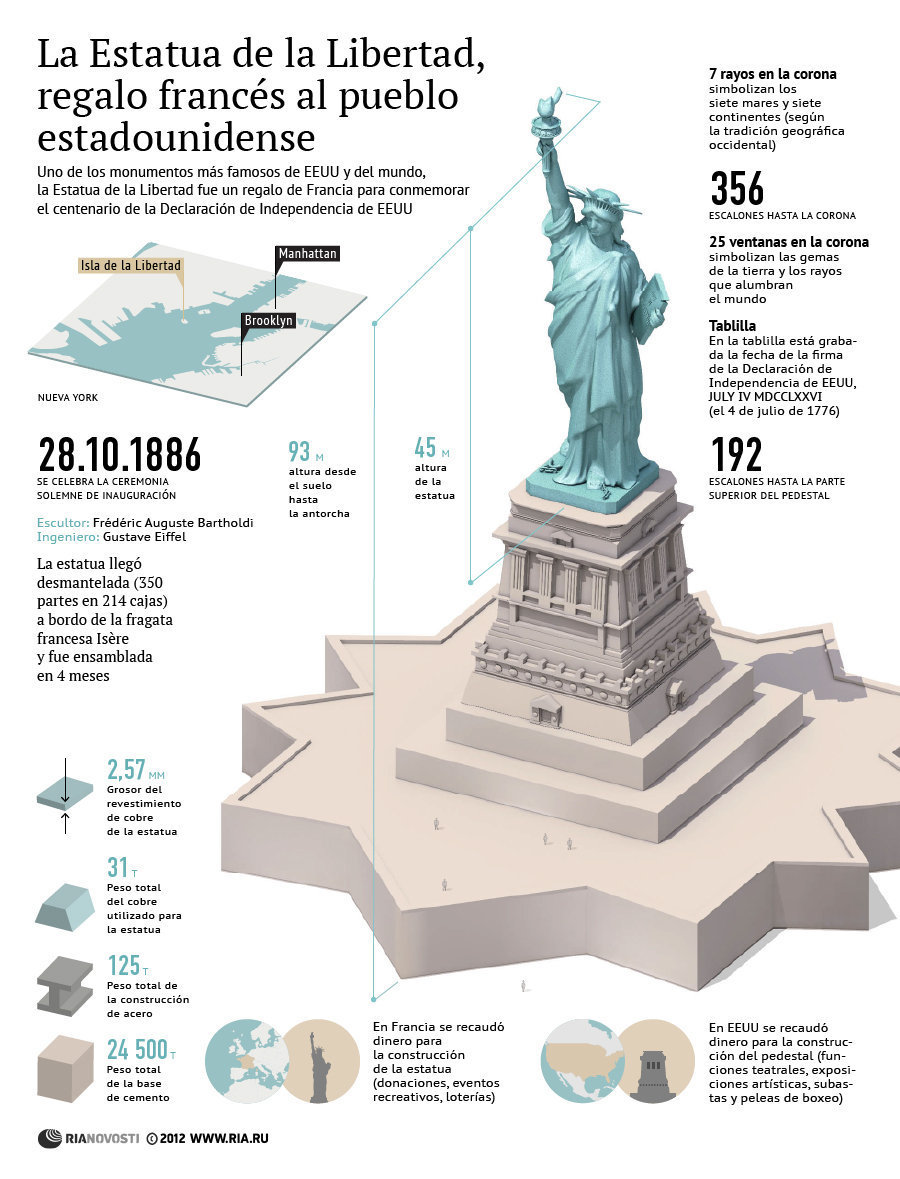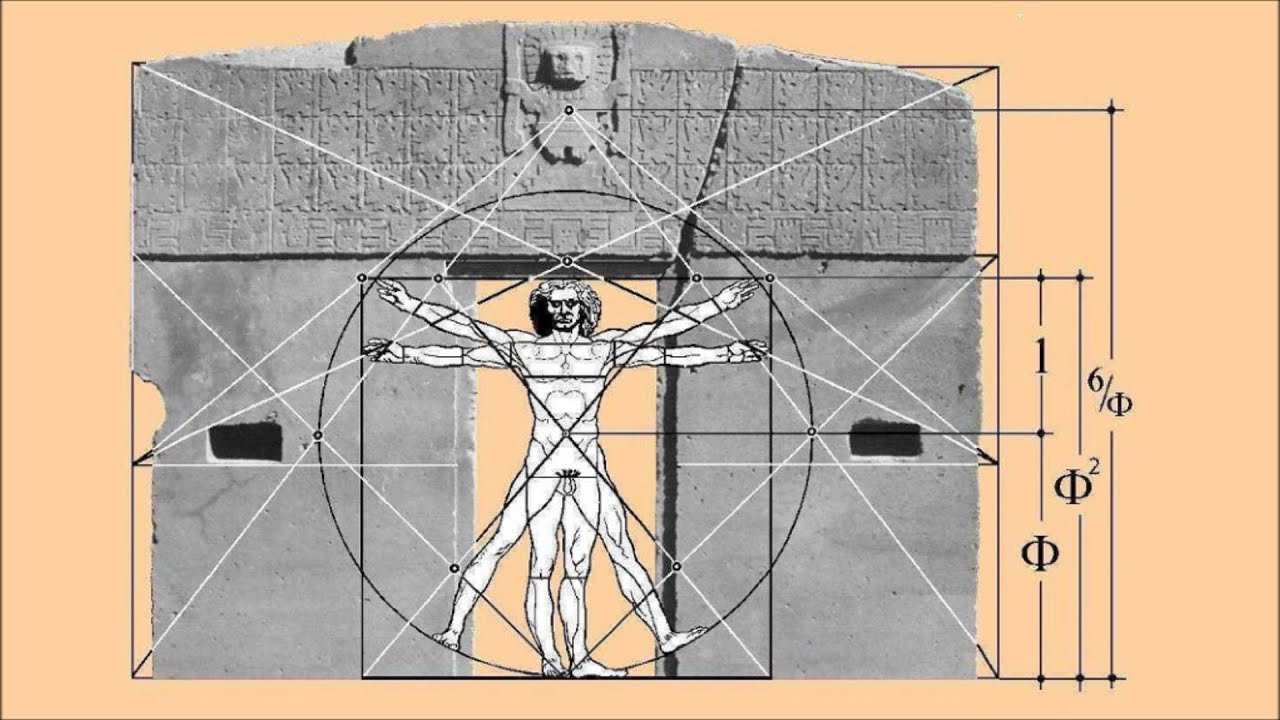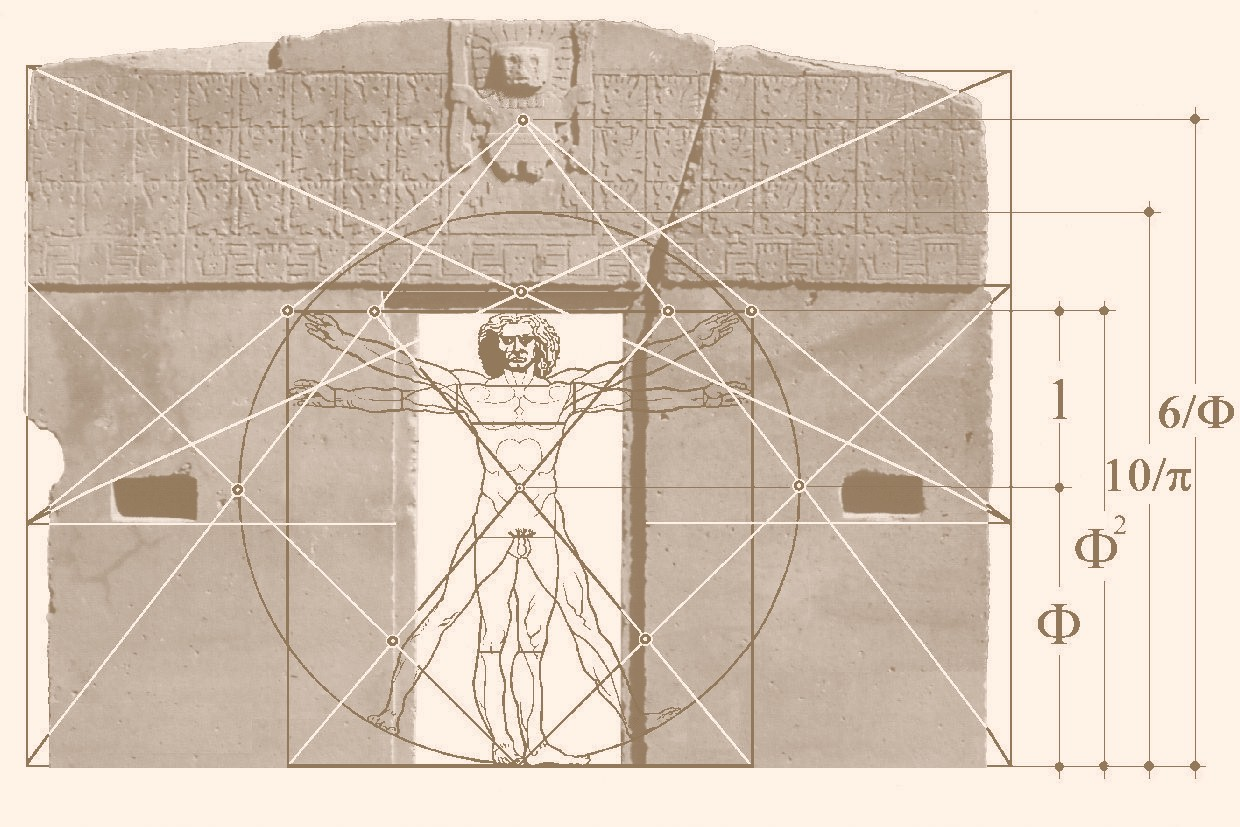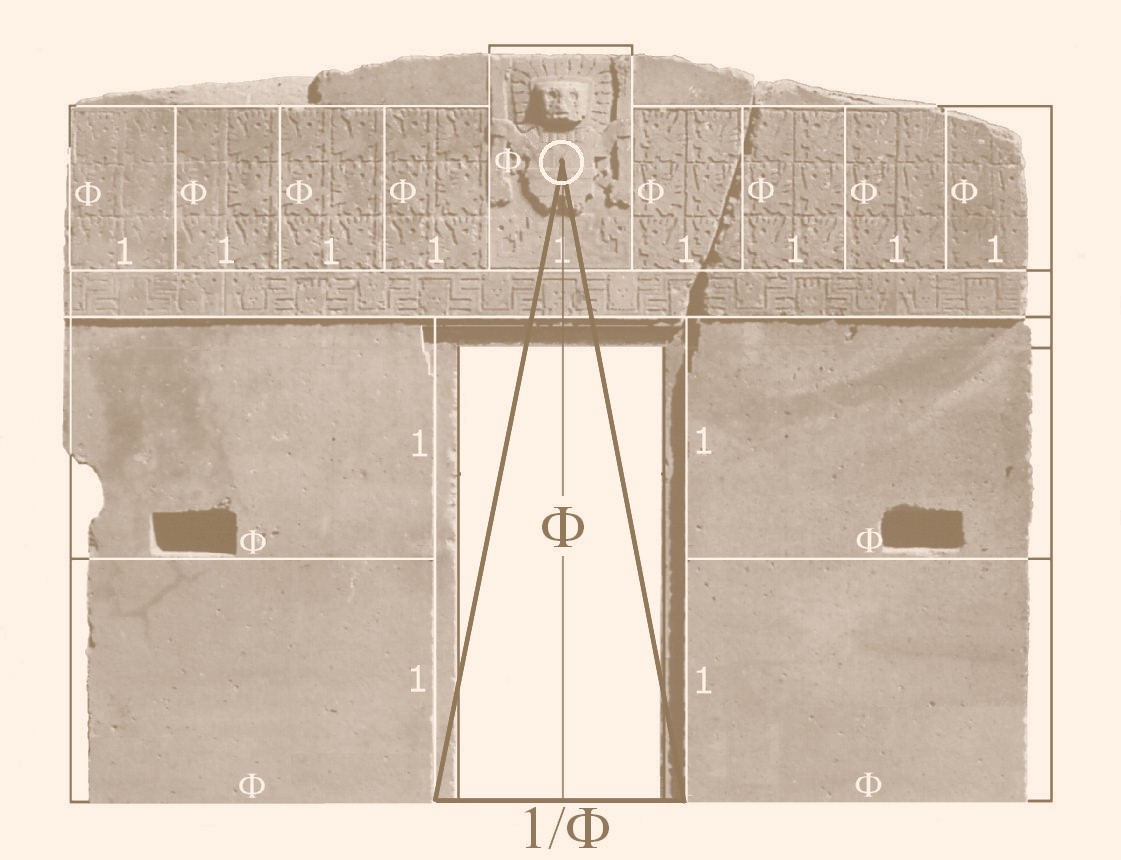LA SANGRE DEL CORDERO EN EL DINTEL, EN CONTEXTO AL EXODO PASCUAL, ES UN TIPO DEL GRIAL
1. Éxodo 12:7: Y tomarán de la sangre, y la pondrán en los dos postes y en el DINTEL de las casas en que lo han de comer.

2. Éxodo 12:22: Y tomad un manojo de hisopo, y mojadlo en la sangre que estará en un lebrillo, y untad el DINTEL y los dos postes con la sangre que estará en el lebrillo; y ninguno de vosotros salga de las puertas de su casa hasta la mañana.

3. Éxodo 12:23: Porque Jehová pasará hiriendo a los egipcios; y cuando vea la sangre en el DINTEL y en los dos postes, pasará Jehová aquella puerta, y no dejará entrar al heridor en vuestras casas para herir.

# Dintel

Saltar a: navegaciónbúsqueda

Un dintel es un elemento estructural horizontal que salva un espacio libre entre dos apoyos. Es el elemento superior que permite abrir huecos en los muros para conformar puertasventanas o pórticos. Por extensión, el tipo de arquitectura, o construcción, que utiliza el uso de dinteles para cubrir los espacios en los edificios se llama arquitectura adintelada, o construcción adintelada. La que utiliza arcos o bóvedas se denomina arquitectura abovedada.

Los mejores exponentes de arquitectura adintelada en piedra son los edificios monumentales del Antiguo Egipto y la Grecia clásica. La palabra dintel proviene de la palabra latina: limitellus, que deriva etimológicamente de limen y limes. En latín la palabra limen significa umbral, puerta, entrada o comienzo, y limes se refiere a un sendero entre dos campos, límite o muralla.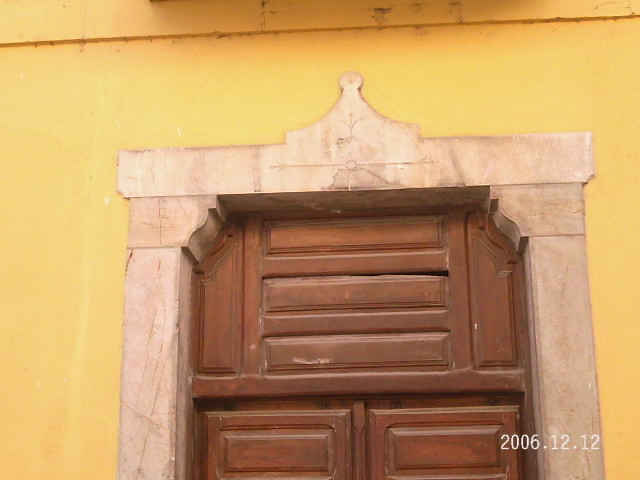Comparen las dos figuras anteriores y noten que la LUZ SOLAR PENETRA, en este caso EN LA PUERTA DE TIWANAKU (BOLIVIA) en los equinoccios, osea el 20/21 de marzo y  los 21/22 de septiembre. CONCRETAMENTE LA FIESTA DE LOS TABERNACULOS ES PRIMA HERMANA DE LA FIESTA PASCUAL. EN ESTE MARCO, INSISTO, EL SOL, SI USTED COMPARA CON LA FIGURA SUPERIOR, EN LOS MISMOS EQUINOCCIOS LA LUZ SOLAR CHOCA CON LA PIRAMIDE VATICANA E INCLUSO INGRESA O PENETRA ADENTRO DEL TEMPLO DE SAN PEDRO. PREGUNTO: ¿SI LA PLAZA DE MARIA DE LA VICTORIA ESTA UBICADA EN LA MISMA LINEA EQUINOCCIAL, QUIEN ES EN ESTE MARCO LA MISMA, EN EL CONTEXTO QUE LA PASCUA TAMBIEN TIENE ESA REFERENCIA? CUALQUIER PERSONA QUE TIENE TRES DEDOS DE FRENTE SE DA CUENTA QUE ES MARIA LA MAGDALENA. SI NO ES ASI PREGUNTO:

¿PORQUE CRISTO SE PRESENTO SIENDO VENCEDOR EL 17 DE NISSAN, OSEA EN EL EQUINOCCIO FRENTE A MARIA MAGDALENA?

CONCLUYO:

MARIA LA VICTORIA ES MARIA MAGDALENA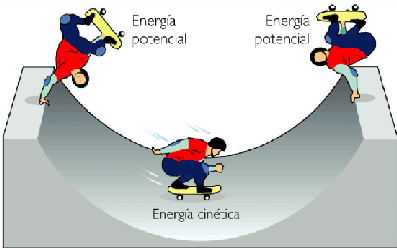Reply Message 72 of 90 on the subject
 From: BARILOCHENSE6999 Sent: 16/09/2021 02:20

 Reply Message 73 of 90 on the subject
 From: BARILOCHENSE6999 Sent: 17/09/2021 00:34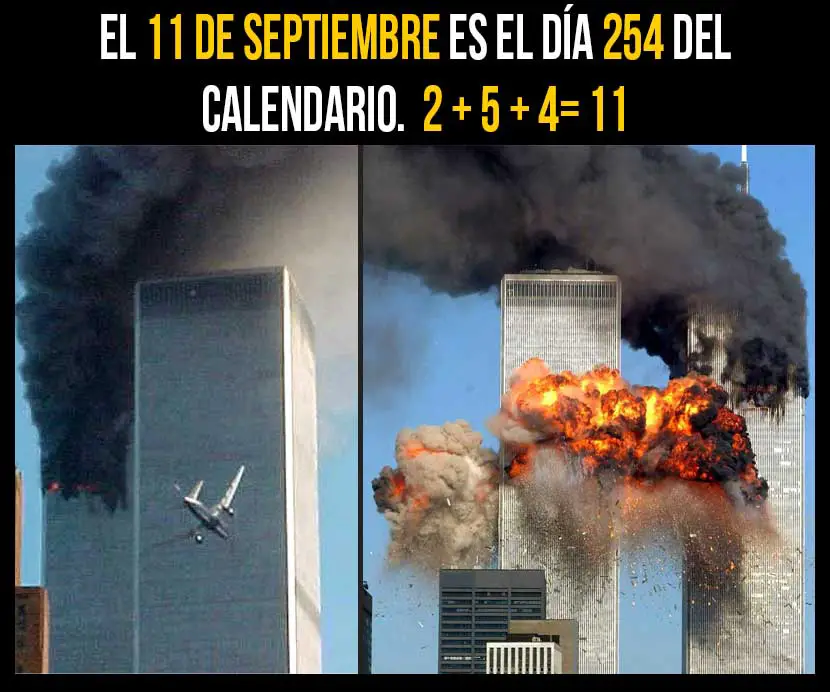The amount of days in a solar year plus the proportions for the Equatorial circumference of the Earth and the proportions of the Great Pyramid of Giza according to Golden Pi = 4/√φ = 3.144605511029693144: The Great Pyramid of Giza is a geodetic model of Planet Earth. The measurements mentioned below are the ideal measurements. A meter is equal to 100 centimeters 1 Solon cubit = 40 times √φ = 50.88078598056276 centimeters. If 1 Solon cubit is divided into 20 equal units of measure then 20 inches can be derived because 1 Solon cubit is equal to 20 inches. 1 Saylen cubit = 50 times √φ = 63.60098247570345 centimeters. If 1 Saylen cubit is divided into 25 equal units of measure then 25 inches can be derived because 1 Saylen cubit is equal to 25 inches. 1 inch = 2 times √φ = 2.544039299028138 centimeters. 1 foot = 12 inches. 1 foot = 24 times √φ = 30.528471588337656 centimeters. If the shortest edge length of a Kepler right triangle is equal to 1 then the hypotenuse of the Kepelr right triangle is equal to The Golden ratio = (√(5) plus 1)/2 = φ = 1.618033988749895 according to the Pythagorean theorem. The Golden ratio in Trigonometry = (cosine (36 degrees) times 2) = 1.618033988749895. If the shortest edge length of a Kepler right triangle is equal to 1 then second longest edge length of the Kepelr right triangle is the square root of the Golden ratio = √φ = 1.272019649514069 according to the Pythagorean theorem. The width for the square base of the Great Pyramid of Giza is equal to 756 feet. • If the shortest edge length of a Kepler right triangle is equal to 1 foot and then the second edge length of the Kepler right triangle is multiplied 378 equal times the result will be the height of the Great Pyramid of Giza = 378 times √φ = 480.823427516318082 feet according to Golden Pi = 4/√φ = 3.144605511029693144. • If the shortest edge length of a Kepler right triangle is equal to 1 foot and then the hypotenuse of the Kepler right triangle is multiplied 378 equal times the result will be the slant height of the Great Pyramid of Giza = 378 times φ = 611.61684774746031 feet according to Golden Pi = 4/√φ = 3.144605511029693144. • If the shorter edge length of a Golden ratio = (√(5) plus 1)/2 = φ = 1.618033988749895 rectangle is equal to 1 foot and then the diagonal of the Golden ratio = (√(5) plus 1)/2 = φ = 1.618033988749895 rectangle is multiplied 378 equal times the result will be the edge height of the Great Pyramid of Giza = 378 times = Cosine (18) degrees times 2 = 1.902113032590307 = 718.998726319136046 feet according to Golden Pi = 4/√φ = 3.144605511029693144.Cosine (18) degrees times 2 = 1.902113032590307. Cosine (18) degrees times 2 = 1.902113032590307 squared = φ plus 2 = 3.618033988749895. • If the shortest edge length of a Kepler right triangle is equal to 1 foot = 24 times √φ = 30.528471588337656 centimeters then the second longest edge length of that Kepler right triangle is equal to 38.832815729997479 centimeters.24 times √φ = 30.528471588337656 centimeters times the square root of the Golden ratio = √φ = 1.272019649514069 = 38.832815729997479 centimeters. 1 foot = 24 times √φ = 30.528471588337656 centimeters times the square root of the Golden ratio = √φ = 1.272019649514069 = 38.832815729997479 centimeters times 378 = the height of the Great Pyramid of Giza of Giza = 14678.804345939047062 centimeters. The height of the Great Pyramid of Giza = 14678.804345939047062 centimeters divided by 24 times √φ = 30.528471588337656 centimeters = the height of the Great Pyramid of Giza = 480.823427516318088 feet. 378 times √φ = 1.272019649514069 = 480.823427516318088. • If the shortest edge length of a Kepler right triangle is equal to 1 foot = 24 times √φ = 30.528471588337656 centimeters then the hypotenuse of that Kepler right triangle is equal to 49.39610465451582 centimeters. 24 times √φ = 30.528471588337656 centimeters times the Golden ratio = (√(5) plus 1)/2 = φ = 1.618033988749895 = 49.39610465451582 centimeters. 1 foot = 24 times √φ = 30.528471588337656 centimeters times the Golden ratio = (√(5) plus 1)/2 = φ = 1.618033988749895 378 = 49.39610465451582 centimeters times 378 = slant the height of the Great Pyramid of Giza = 18671.727559406979971centimeters. The Slant height of the Great Pyramid = 18671.727559406979971 centimeters divided by 24 times √φ = 30.528471588337656 centimeters = the slant height of the Great Pyramid of Giza = 611.61684774746031 feet. 378 times √φ = 1.272019649514069 = 611.61684774746031. • If the shortest edge length of a Golden ratio = (√(5) plus 1)/2 = φ = 1.618033988749895 rectangle is equal to 1 foot = 24 times √φ = 30.528471588337656 centimeters then the length of the diagonal of that Golden ratio = (√(5) plus 1)/2 = φ = 1.618033988749895 rectangle is equal to 58.068603673239965 centimeters. 24 times √φ = 30.528471588337656 centimeters times Cosine (18) degrees times 2 = 1.902113032590307 = 58.068603673239965 centimeters. Cosine (18) degrees times 2 = 1.902113032590307 squared = φ plus 2 = 3.618033988749895. 1 foot = 24 times √φ = 30.528471588337656 centimeters times = Cosine (18) degrees times 2 = 1.902113032590307 = 58.068603673239965 centimeters times 378 = the edge height of the Great Pyramid of Giza of Giza = 21949.932188484706835 centimeters. The edge height of the Great Pyramid = 21949.932188484706835 centimeters divided by 24 times √φ = 30.528471588337656 centimeters = the edge height of the Great Pyramid of Giza = 718.998726319136046 feet. 378 times Cosine (18) degrees times 2 = 1.902113032590307 = 718.998726319136046. Cosine (18) degrees times 2 = 1.902113032590307. Cosine (18) degrees times 2 = 1.902113032590307 squared = φ plus 2 = 3.618033988749895. A Kepler right triangle can be created from the construction of a Golden ratio = (√(5) plus 1)/2 = φ = 1.618033988749895 rectangle by using Compass and straight edge and obviously a marker for the drawing surface. The amount of days in a Solar year = 4/√φ times 7920 times 5280/(10 ^ 3 times 360) = 365.277376161209156. The amount of days in a Solar year = 4/√φ = 3.144605511029693144 times 7920 times 5280/(10 ^ 3 times 360) = 365.277376161209156. The equatorial circumference of planet Earth = 10 ^ 3 times 360 times 365.277376161209156 = 131499855.41803529616 feet. The equatorial circumference of planet Earth = 4/√φ = 3.144605511029693144 times 7920 = 24905.275647355169727 statute miles. 131499855.41803529616 feet divided by 86400 = half the perimeter of the socle of the Great Pyramid of Giza = 1521.989067338371483 feet. Half the perimeter of the socle of the Great Pyramid of Giza times 86400 is also equal to the equatorial circumference of planet Earth = 131499855.41803529616 feet. 484 divided by √φ times 2 = the width of the socle of the Great Pyramid of Giza = 760.99453366918572 feet. Half the width of the socle of the Great Pyramid of Giza = 380.49726683459286 feet times √φ = 484 feet. 484/√φ times 2 times 2 times 86400 = 131499855.41803529616 feet. 131499855.41803529616 feet divided by 5280 = The perimeter of the socle of the Great Pyramid of Giza = 484/√φ times 8 = 3043.978134676742966 feet. 484 feet /√φ times 8 = 3043.978134676742966 feet times 12 = 24905.275647355169727 statute miles. 24905.275647355169727 statute miles divided by 7920 statute miles = Golden Pi = 4/√φ = 3.144605511029693144. There are 929.28 meters in the square perimeter of the socle of the Great Pyramid of Giza according to Golden Pi = 4/√φ = 3.144605511029693144. 484/√φ times 8 = 3043.978134676742966 times 12 = 36527.737616120915592 divided by 100 = the exact amount of days in a solar year = 365.277376161209156. The equatorial diameter of our planet Earth = 41817600 feet. 41817600 feet divided 86400 = the height of the Great Pyramid of Giza = 378 times √φ = 480.823427516318082 feet plus the height of the socle of the Great Pyramid of Giza = 3.176572483681918 feet. 10 ^ 3 times 360 times 484/(√φ) times 8 times 12/(100) = 131499855.41803529616 feet. The height of the Great Pyramid if Giza is 378 times √φ = 480.823427516318082 feet. The width of the square base of the Great Pyramid of Giza is 756 feet. The perimeter of the square base of the Great Pyramid of Giza = 3024 feet. 9 factorial = 362880. At 10 degrees latitude the length of a degree is 9 factorial =362880 feet. The amount of inches in the perimeter of the square of the Great Pyramid of Giza = 36288. 36288 times 10 = 362880. The width for the square base of the Great Pyramid of Giza = 756 feet. The perimeter of the square base of the Great Pyramid of Giza = 3024 feet. There are 36288 inches in the perimeter of the square base of the Great Pyramid of Giza. 3024 times 12 = 36288. 756 times 4 times 12 = 36288. (9!)/10 = 36288. The equatorial circumference of planet Earth: https://joedubs.com/four-earthly-elements/equatorial-circumference-of-earth/ Kepler right triangle diagram with squares upon the edges of the Kepler right triangle: https://drive.google.com/file/d/1iBtXYy06yv9UWtGP5mwMXyt80vaysFvR/view?usp=sharing Kepler right triangle construction method: https://drive.google.com/file/d/15DNXB_xNP2f2jCroC0FyNUyBYGhVAA2J/view?usp=sharing PYTHAGOREAN THEOREM: https://en.wikipedia.org/wiki/Pythagorean_theorem Golden ratio: https://en.wikipedia.org/wiki/Golden_ratio The history of the meter: The history of the meter: https://www.factinate.com/editorial/meter-history/ The meter: https://en.wikipedia.org/wiki/Metre The meter is based now on the speed of light: https://www.youtube.com/watch?v=vgqUyFaUDcI

 Respuesta Mensaje 13 de 14 en el temaRespuesta Mensaje 14 de 14 en el temaFirstPrevious  61 a 75 de 90  NextLastPrevious subject  Next subject